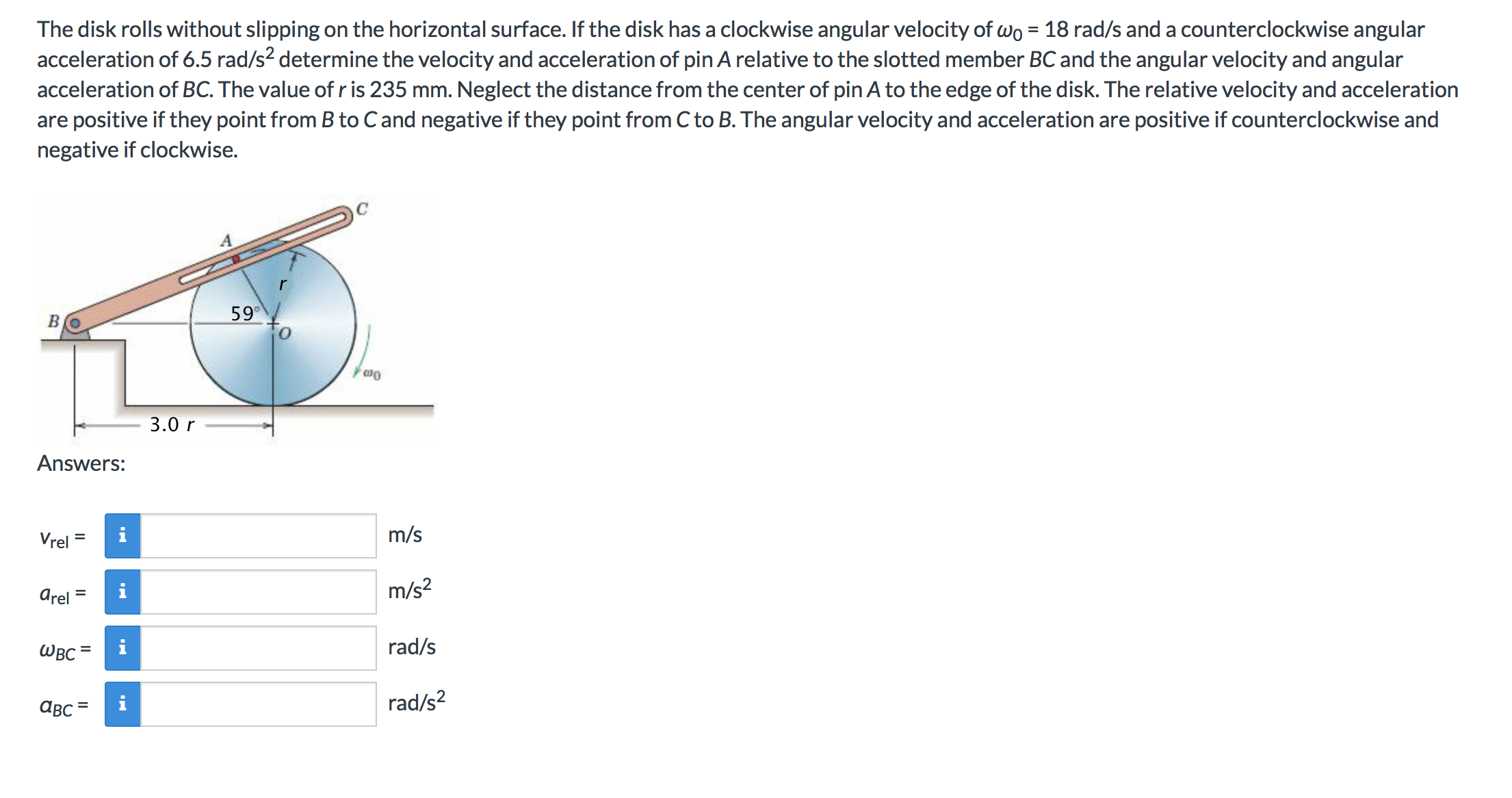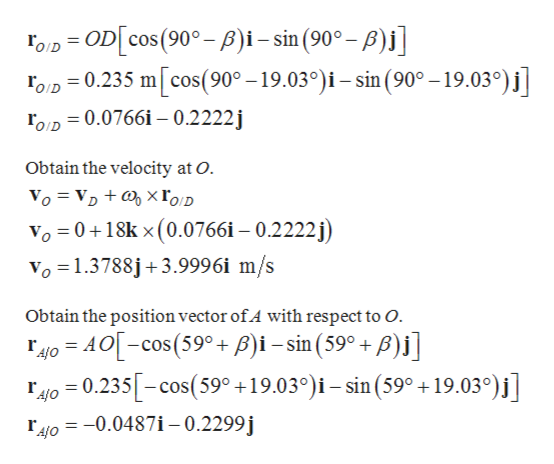# The disk rolls without slipping on the horizontal surface. If the disk has a clockwise angular velocity of wo 18 rad/s and a counterclockwise angularacceleration of 6.5 rad/s2 determine the velocity and acceleration of pin A relative to the slotted member BC and the angular velocity and angularacceleration of BC. The value of r is 235 mm. Neglect the distance from the center of pin A to the edge of the disk. The relative velocity and accelerationare positive if they point from B to Cand negative if they point from C to B. The angular velocity and acceleration are positive if counterclockwise andnegative if clockwise.59Om3.0 rAnswers:m/siVrelm/s2arelrad/sШвсrad/s2авсIl

Questionhelp_outlineImage TranscriptioncloseThe disk rolls without slipping on the horizontal surface. If the disk has a clockwise angular velocity of wo 18 rad/s and a counterclockwise angular acceleration of 6.5 rad/s2 determine the velocity and acceleration of pin A relative to the slotted member BC and the angular velocity and angular acceleration of BC. The value of r is 235 mm. Neglect the distance from the center of pin A to the edge of the disk. The relative velocity and acceleration are positive if they point from B to Cand negative if they point from C to B. The angular velocity and acceleration are positive if counterclockwise and negative if clockwise. 59 Om 3.0 r Answers: m/s i Vrel m/s2 arel rad/s Швс rad/s2 авс Il fullscreen
check_circle

Step 1

Draw the triangle formed by joining the points OAB.

Step 2

Apply sine rule.help_outlineImage TranscriptioncloseOA ОВ sin 180° - (B+59) sin B 3r sin B sinB 59°) 1 3 sin B sin Bcos59° + cos Bsin 59° 1 sin B 0.515 sinB+0.857 cos B 0.515 0.857 cotB = 3 B 19.03° Obtain the distance between A and B АВ OA sin B sin 59° 0.235 m АВ sin 59° sin 19.03° AB 0.6178 m fullscreen
Step 3

Obtain the position vector of O with respe...help_outlineImage TranscriptioncloseFoD= OD[cos(90°-)i-sin (90°- p)]] roD 0.235 m[cos(90°-19.03°)i- sin (90° -19.03°)j] roD 0.0766i 0.2222j Obtain the velocity at O Vo- VD + 0 xгоD Vo 0+18k x (0.0766i - 0.2222j Vo 1.3788j+3.9996i m/s Obtain the position vector of.4 with respect to O 4o AO-cos(59+ P)i-sin(59° + B)j] r4o 0.235-cos(59° +19.03°)i-sin (59° +19.03°)j] o0.0487i -0.2299j fullscreen

### Want to see the full answer?

See Solution

#### Want to see this answer and more?

Solutions are written by subject experts who are available 24/7. Questions are typically answered within 1 hour.*

See Solution
*Response times may vary by subject and question.
Tagged in

### Mechanical Engineering